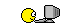Dan Schmidt - your answer is right! But now I'm not in the mood for talking math until we get the LaTeX fixed.Joel Sjögren - I will answer your question sometime, but right now I'm just trying to see if LaTeX works here. For some reason Dan's equations didn't work. My own equation, `\$\$E = mc^2\$\$`, didn't work _at first_, but now mysteriously it has!

I need to do some testing.

This: `\$E = mc^2\$`

yields this: \$E = mc^2\$

This: `\(E= mc^2\)`

yields this: \(E = mc^2\)

This: `\$\$E = mc^2\$\$`

yields this: \$\$E = mc^2\$\$

This: `\[E = mc^2\]`

yields this: \[E= mc^2\]